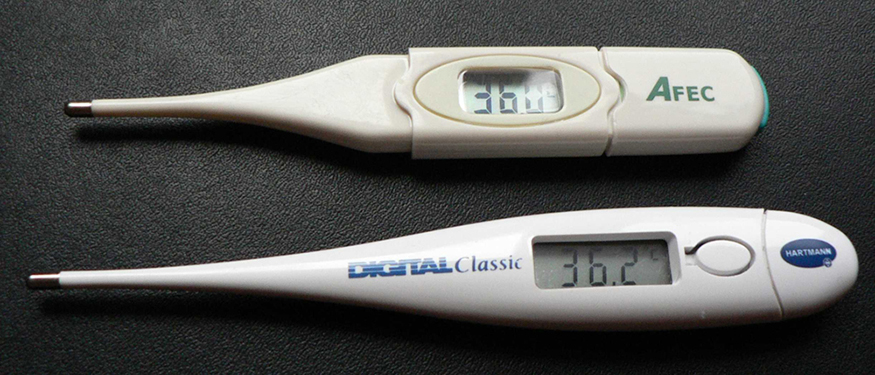# 20.3 Resistance and resistivity  (Page 3/6)

 Page 3 / 6

The resistance of an object also depends on temperature, since ${R}_{0}$ is directly proportional to $\rho$ . For a cylinder we know $R=\mathrm{\rho L}/A$ , and so, if $L$ and $A$ do not change greatly with temperature, $R$ will have the same temperature dependence as $\rho$ . (Examination of the coefficients of linear expansion shows them to be about two orders of magnitude less than typical temperature coefficients of resistivity, and so the effect of temperature on $L$ and $A$ is about two orders of magnitude less than on $\rho$ .) Thus,

$R={R}_{0}\left(\text{1}+\alpha \Delta T\right)$

is the temperature dependence of the resistance of an object, where ${R}_{0}$ is the original resistance and $R$ is the resistance after a temperature change $\Delta T$ . Numerous thermometers are based on the effect of temperature on resistance. (See [link] .) One of the most common is the thermistor, a semiconductor crystal with a strong temperature dependence, the resistance of which is measured to obtain its temperature. The device is small, so that it quickly comes into thermal equilibrium with the part of a person it touches.These familiar thermometers are based on the automated measurement of a thermistor’s temperature-dependent resistance. (credit: Biol, Wikimedia Commons)

## Calculating resistance: hot-filament resistance

Although caution must be used in applying $\rho ={\rho }_{0}\left(\text{1}+\alpha \Delta T\right)$ and $R={R}_{0}\left(\text{1}+\alpha \Delta T\right)$ for temperature changes greater than $\text{100º}\text{C}$ , for tungsten the equations work reasonably well for very large temperature changes. What, then, is the resistance of the tungsten filament in the previous example if its temperature is increased from room temperature ( $\text{20ºC}$ ) to a typical operating temperature of $\text{2850º}\text{C}$ ?

Strategy

This is a straightforward application of $R={R}_{0}\left(\text{1}+\alpha \Delta T\right)$ , since the original resistance of the filament was given to be ${R}_{0}=0\text{.}\text{350 Ω}$ , and the temperature change is $\Delta T=\text{2830º}\text{C}$ .

Solution

The hot resistance $R$ is obtained by entering known values into the above equation:

$\begin{array}{lll}R& =& {R}_{0}\left(1+\alpha \Delta T\right)\\ & =& \left(0\text{.}\text{350 Ω}\right)\left[\text{1}+\left(4.5×{\text{10}}^{–3}/\text{ºC}\right)\left(\text{2830º}\text{C}\right)\right]\\ & =& \text{4.8 Ω.}\end{array}$

Discussion

This value is consistent with the headlight resistance example in Ohm’s Law: Resistance and Simple Circuits .

## Phet explorations: resistance in a wire

Learn about the physics of resistance in a wire. Change its resistivity, length, and area to see how they affect the wire's resistance. The sizes of the symbols in the equation change along with the diagram of a wire.

## Section summary

• The resistance $R$ of a cylinder of length $L$ and cross-sectional area $A$ is $R=\frac{\mathrm{\rho L}}{A}$ , where $\rho$ is the resistivity of the material.
• Values of $\rho$ in [link] show that materials fall into three groups— conductors, semiconductors, and insulators .
• Temperature affects resistivity; for relatively small temperature changes $\Delta T$ , resistivity is $\rho ={\rho }_{0}\left(\text{1}+\alpha \Delta T\right)$ , where ${\rho }_{0}$ is the original resistivity and $\text{α}$ is the temperature coefficient of resistivity.
• [link] gives values for $\alpha$ , the temperature coefficient of resistivity.
• The resistance $R$ of an object also varies with temperature: $R={R}_{0}\left(\text{1}+\alpha \Delta T\right)$ , where ${R}_{0}$ is the original resistance, and $R$ is the resistance after the temperature change.

## Conceptual questions

In which of the three semiconducting materials listed in [link] do impurities supply free charges? (Hint: Examine the range of resistivity for each and determine whether the pure semiconductor has the higher or lower conductivity.)

What is the difference between a principle and a law
the law is universally proved. The principal depends on certain conditions.
Dr
what does the speedometer of a car measure ?
Car speedometer measures the rate of change of distance per unit time.
Moses
describe how a Michelson interferometer can be used to measure the index of refraction of a gas (including air)
using the law of reflection explain how powder takes the shine off a person's nose. what is the name of the optical effect?
WILLIAM
is higher resolution of microscope using red or blue light?.explain
WILLIAM
what is dimensional consistent
Mohammed
In engineering and science, dimensional analysis is the analysis of the relationships between different physical quantities by identifying their base quantities and units of measure and tracking these dimensions as calculations or comparisons are performed
syed
can sound wave in air be polarized?
Unlike transverse waves such as electromagnetic waves, longitudinal waves such as sound waves cannot be polarized. ... Since sound waves vibrate along their direction of propagation, they cannot be polarized
Astronomy
A proton moves at 7.50×107m/s perpendicular to a magnetic field. The field causes the proton to travel in a circular path of radius 0.800 m. What is the field strength?
derived dimenionsal formula
what is the difference between mass and weight
assume that a boy was born when his father was eighteen years.if the boy is thirteen years old now, how is his father in
Isru
what is airflow
derivative of first differential equation
why static friction is greater than Kinetic friction
draw magnetic field pattern for two wire carrying current in the same direction
An American traveler in New Zealand carries a transformer to convert New Zealand’s standard 240 V to 120 V so that she can use some small appliances on her trip.
What is the ratio of turns in the primary and secondary coils of her transformer?
nkombo
what is energy
Yusuf
How electric lines and equipotential surface are mutually perpendicular?
The potential difference between any two points on the surface is zero that implies È.Ŕ=0, Where R is the distance between two different points &E= Electric field intensity. From which we have cos þ =0, where þ is the angle between the directions of field and distance line, as E andR are zero. Thus
sorry..E and R are non zero...

#### Get Jobilize Job Search Mobile App in your pocket Now!ByByBy Jonathan LongByBy RhodesBy OpenStaxBy Savannah ParrishBy Rohini AjayBy Mary MateraBy Rachel WoolardBy Yacoub JayoghliBy Qqq Qqq